# Section 9 2 Sampling Proportions AP Statistics Section

• Slides: 13Section 9. 2 Sampling Proportions AP Statistics, Section 9. 2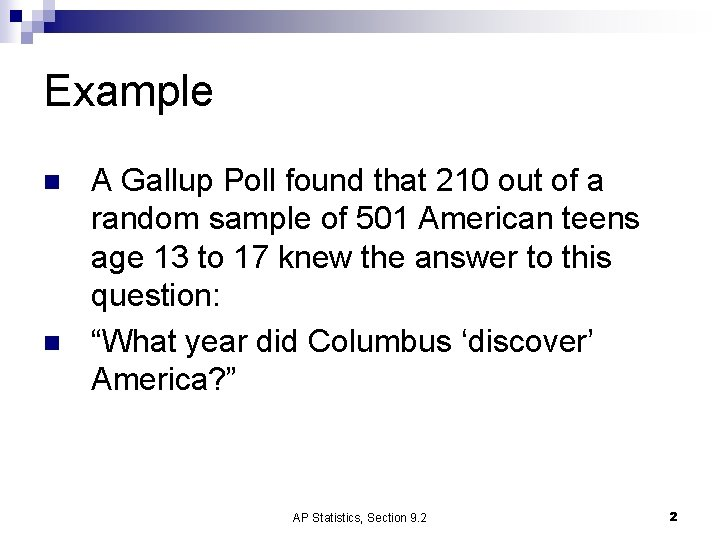Example n n A Gallup Poll found that 210 out of a random sample of 501 American teens age 13 to 17 knew the answer to this question: “What year did Columbus ‘discover’ America? ” AP Statistics, Section 9. 2 2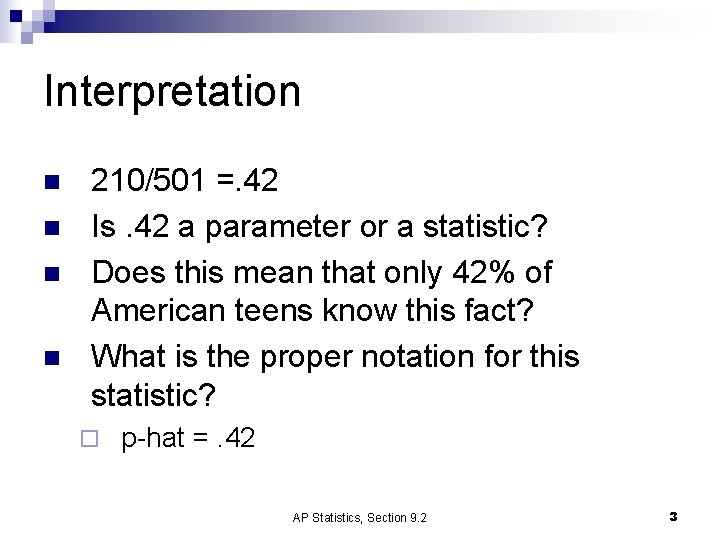Interpretation n n 210/501 =. 42 Is. 42 a parameter or a statistic? Does this mean that only 42% of American teens know this fact? What is the proper notation for this statistic? ¨ p-hat =. 42 AP Statistics, Section 9. 2 3New Formulas AP Statistics, Section 9. 2 4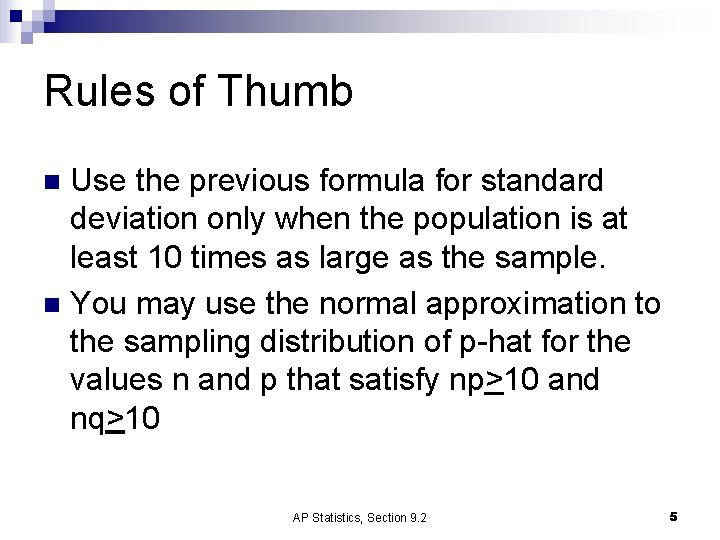Rules of Thumb Use the previous formula for standard deviation only when the population is at least 10 times as large as the sample. n You may use the normal approximation to the sampling distribution of p-hat for the values n and p that satisfy np>10 and nq>10 n AP Statistics, Section 9. 2 5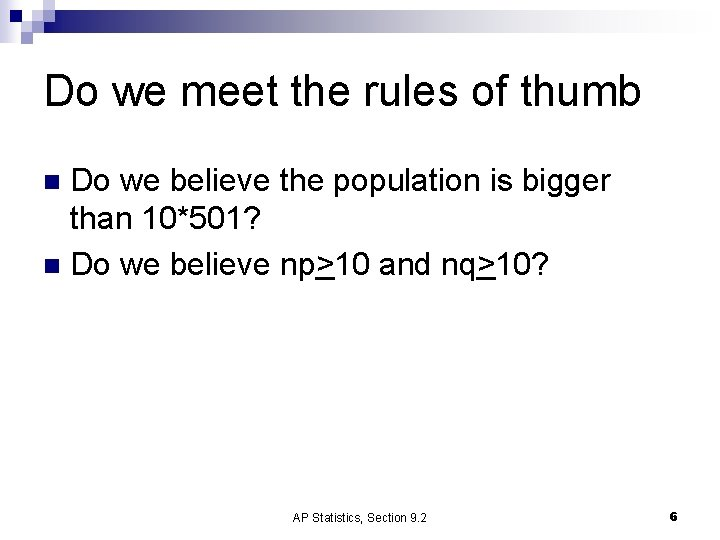Do we meet the rules of thumb Do we believe the population is bigger than 10*501? n Do we believe np>10 and nq>10? n AP Statistics, Section 9. 2 6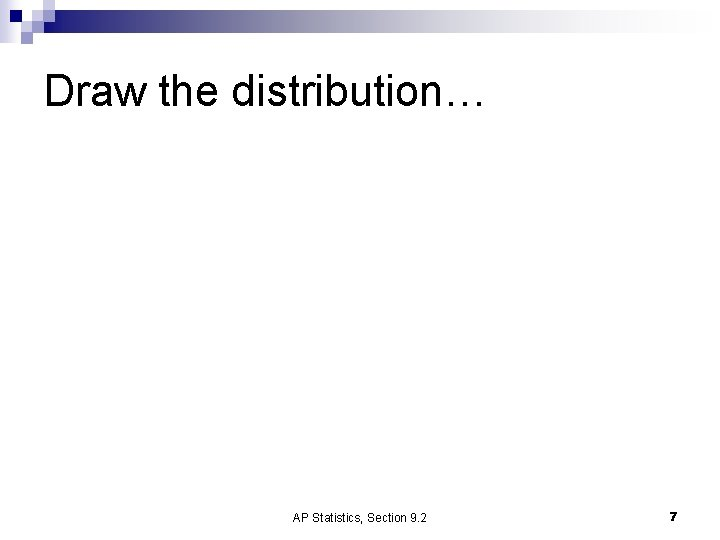Draw the distribution… AP Statistics, Section 9. 2 7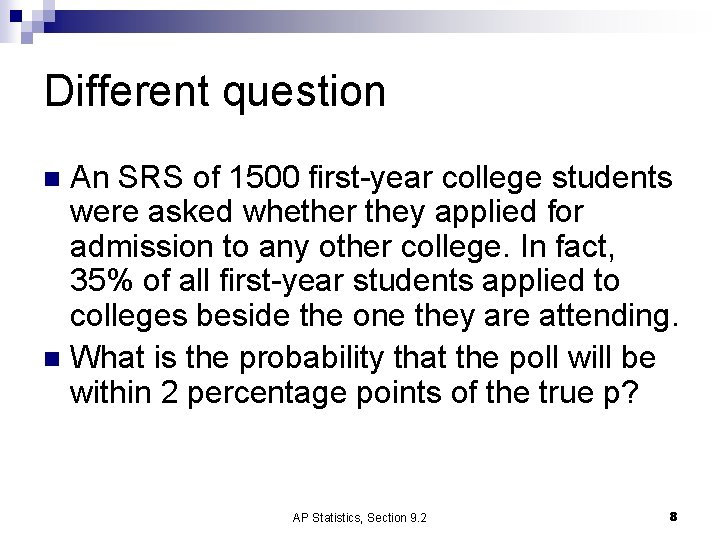Different question An SRS of 1500 first-year college students were asked whether they applied for admission to any other college. In fact, 35% of all first-year students applied to colleges beside the one they are attending. n What is the probability that the poll will be within 2 percentage points of the true p? n AP Statistics, Section 9. 2 8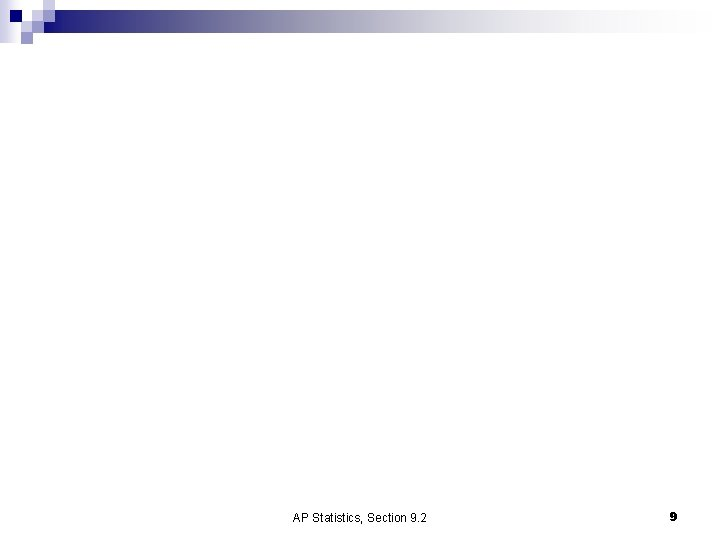AP Statistics, Section 9. 2 9Conclusion n About 90% of the samples fall within 2% of the real p. AP Statistics, Section 9. 2 10Another example n n n One way of checking the effect of undercoverage, nonresponse, and other sources of error in a sample survey is to compare the sample with known facts about the population. About 11% of American adults are black. The proportion p-hat in an SRS should be about. 11. If a national sample contains only 9. 2% blacks, should we suspect nonresponse bias? AP Statistics, Section 9. 2 11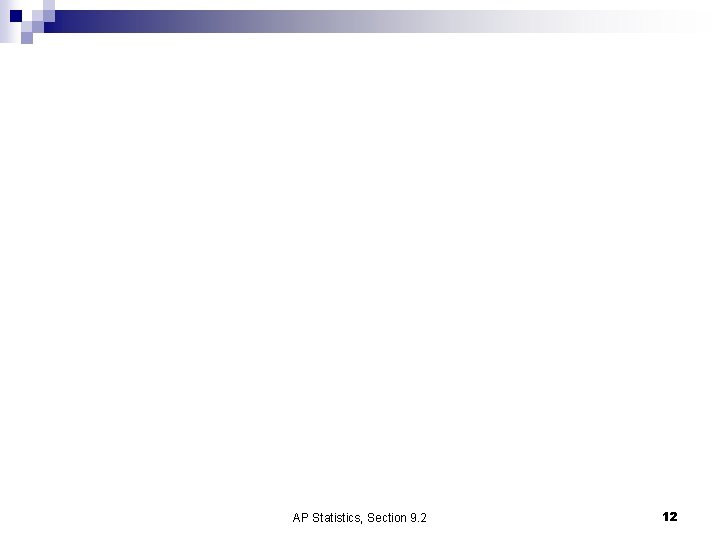AP Statistics, Section 9. 2 12Exercises n 9. 19 -9. 29 odd AP Statistics, Section 9. 2 13